# Markov Random Field Model in Machine Learning

In this tutorial, we will learn about the Markov random field model in Machine Learning. By Bharti Parmar Last updated : April 17, 2023

## What is Markov Random Field Model?

The Markov Random Field model is a model which use an undirected graph. Undirected graphical models edge represents the potential between two variables, syntactically, Factorization distribution probabilities between variable. In each Individual variable connected with the edge represent a certain clique in the graph; means probability distribution of the variables in the graph can factorize an individual clique potential function.

Just as we had CPDs for Bayesian networks, we have tables to incorporate relations between nodes in Markov networks. However, there are two crucial differences between these tables and CPDs.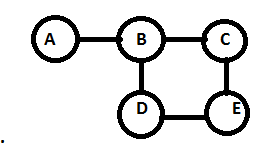Clique in graph theory.it is a subset of vertices of an undirected graph.

P(A, B, C, D, E) α Ф(A,B) Ф (B,C) Ф (B,D) Ф (C,E) Ф (D,E)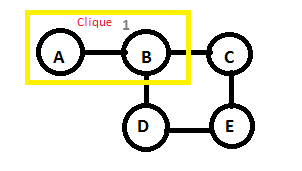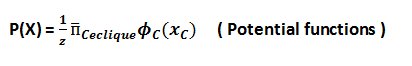Such that: It induces sub graph is complete in every vertices in a clique is adjacent. So, clique in this graph adjust adjacently one by one.

Like,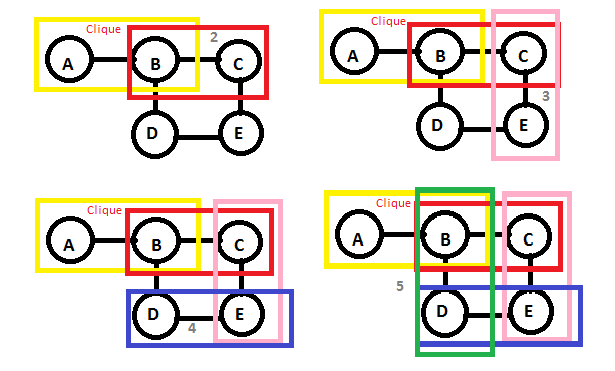It is some different if we join D,C and B,E clique over here then it is also change its probability.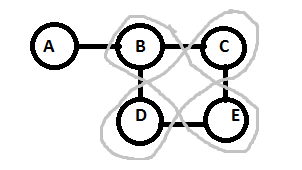P(A, B, C, D, E) α Ф(A,B) Ф (B,C,D) Ф (C,D,E)

Some undirected graphic model has Markov Random Field. In MRF certain paths between A and C.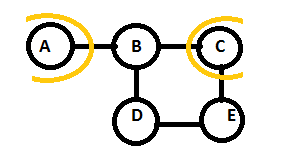```	A -> B -> C
A -> B -> D -> E -> C
```

Note that: Markov networks do not need to be acyclic, as was the case with Bayesian networks.

Independence properties such as Markov properties:

• Any 2 subsets if variables are conditionally independent given a separating subset.
• If we take 'A' as a subset and 'C' as one subset then there is wore between them. So, there is no way to go between 'A' and 'C' without getting threw the subset. So, we are using (A, B) than B, C, D, E.

Therefore, A and C are separating subsets

• Any 2 subset of variable are conditionally independent given a separating subset.
• {B,D}, {B,E} and {B,D,E} are separating subsets.